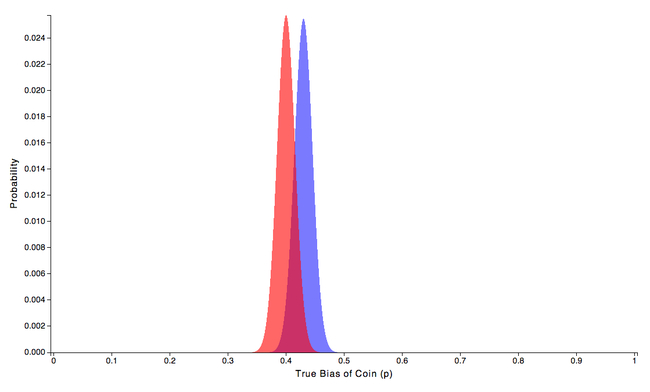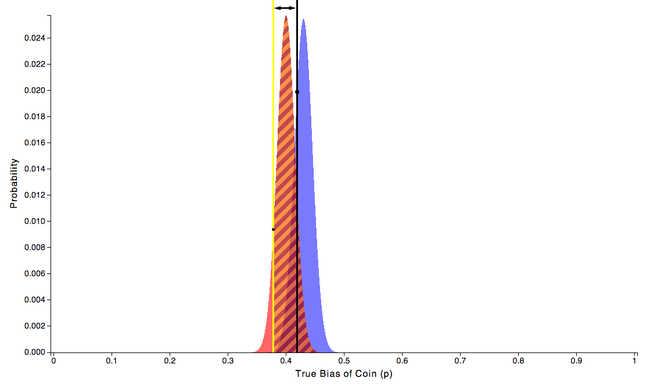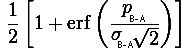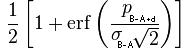# The Math of Split Testing Part 3: The Chance of being Similar

tl;dr

Sometimes we want to verify that a new design will convert at nearly the same rate as an old design. Split tests of this type are not intended to find conversion rate wins, but rather to ensure that the new design is not “too much worse” than the old design. Here, we demonstrate how to analyze a split test of this sort and present an approximate formula to quickly calculate the chance that there is an acceptable difference between the conversion rates of the new and old designs.

Let’s say that we think that the design of our website is starting to look a little outdated and needs to be redone. In this scenario, we’re not really looking to increase conversion rate. We’d be happy to keep our current conversion rate and just make the website look a little more hip. To verify that our new design (branch B) converts at a similar rate as our old design (branch A) we should run a split test.

First, we need to formalize what we mean by “converts at a similar rate” by defining a cutoff value. This cutoff value can be whatever we want. For example, let’s say that we’d be happy if the conversion rate difference between branch B and branch A was no worse than 10%. In this case, our cutoff value would be ten percent of the conversion rate of branch A. Once this cutoff value has been defined, we can proceed to examine the results of our split test.

Let’s assume that the data we collect during our split test indicates that the conversion rate of our new design is worse than our old design, but still within our tolerance. We now need to answer the question “Is the difference between the conversion rates of branch B and branch A really within our tolerance, or is it just a fluke?” After all, It is possible that branch B is much worse than branch A and the fact that it looks the same is due to chance. Maybe branch B just happened to get a few good visitors and branch A randomly got a few worthless ones.

To make things a little more concrete let’s say that we collect 1000 data points for each branch and that 430 people converted on our original design (branch A), shown in blue, but only 400 people convert on our snazzy new design (branch B), shown in red.We calculate the conversion rates to be

pA = 0.43

pB = 0.4

and the conversion rate difference to be

(pB - pA) / pA = 0.03 / 0.43 = 0.069767

So there is about a 7% difference between the branches. We note that this 7% difference is within our 10% tolerance. In Part 2: The Chance to being Better, we answered the question “Is branch B really worse than branch A or is it just a fluke that we happened to measure it that way?” Doing the appropriate calculation presented there yields that there is a 91.34% chance that branch B is worse than branch A. However, that isn’t the question we’re currently after. What we want to know is how likely it is that our measured conversion rate for branch B of 0.4 is not “too much worse”, meaning the we want to make sure there is a high likelihood that branch B is not more than 10% worse than branch A.

To conceptualize the problem we going to look at the process in two steps. In the first step, we choose an arbitrary true coin bias for branch A, for example, lets choose 0.42. Next, we look to see what portion of branch B falls above our threshold. In our case, 10% of branch A’s expected conversion rate is 0.1 * 0.43 = 0.043, so we need to move over from our arbitrarily chosen branch A value by that amount 0.42 - 0.043 = 0.377, and calculate the probability that branch B falls above the offset value 0.377.Finding this probability tell us the chance that branch B is above the 10% worse cutoff for the chosen branch A value of 0.42. The second step of our two step process is to repeat the same calculation for every other possible value of branch A and add them together, weighing each individual contribution by the probability of occurrence of that specific branch A value. Adding together all of the individual contributions for every possible branch A value gives us the total chance that branch B is better than our 10% threshold.

Using a computer to brute force the computation, we find that there is a 71.6% chance that the conversion rate of branch B is above of our 10% limit.

To summarize the results of our split test, we’ve found that:

• Branch B is expected to be 7% worse than branch A
• There is a 91.34% chance that branch B is worse than branch A
• There is a 71.6% chance that the conversion rate difference between branch B and branch A is not worse than 10%

The important insight to take away from these results is that while we’re doing quite well determining if branch A is better than branch B, we are doing quite poorly figuring out if the conversion rate difference between branch B and branch A is not worse than 10%. If we want to become more certain, we’re going to have to take more data. If we assume that conversion rates of branch A and branch B remain the same as we take more data, then we can produce the following table:

 Number of Samples per Branch Chance that the Conversion Rate Difference between Branch B and Branch A is Not Worse than 10% 1000 71.6% 2000 78.9% 3000 83.7% 4000 87.2% 5000 89.8% 6000 91.8% 7000 93.4% 8000 94.6%

While our brute force numerical approach to generate these numbers works just fine, it would be nice to have a simple approximate formula we could use instead. As we discussed in Part 2, it is possible to think of the problem the other way around, and examine the true coin bias of the difference between the branches (B - A) instead of comparing the true coin biases of each branch.

Although the situation is different this time, all we have to do to compensate for the fact that we now have to incorporate a tolerance is to make one small change. From Part 2, we had that the chance that B is better than A is given by:with true coin bais (p)

pB-A = pB - pA

and standard deviation (σ)

σ2B-A = σ2B + σ2A

where erf is the error function.

The only thing we need to change to account for the fact that we now have a tolerance is to include it in the difference of true coin biases. If we call the tolerance bound, d, then we have:

pB-A+d = pB - pA + d

For example, in the case above, our tolerance bound d would be given by

d = percent tolerance * pA = 0.1 * 0.43 = 0.043

Thus, the formula to compute the chance that the true coin bias of branch B is not worse than a lower bound d referenced from the expected true coin bias of branch A is given by:As a check, we use this approximate formula to generate the same table as above

 Number of Samples per Branch Chance that the Conversion Rate Difference between Branch B and Branch A is Not Worse than 10% 1000 72.2% 2000 79.8% 3000 84.7% 4000 88.1% 5000 90.1% 6000 92.6% 7000 94.1% 8000 95.2%

Inspecting the table we can see that these approximate results agree with our brute force calculation to within a percent. One note of caution, just as we mentioned in Part 2, care must be taken to ensure that a Gaussian approximation is valid before using this numerical shortcut.

In summary, with only a slight modification to the equations we used in Part 2, we are now able to find the chance that the conversion rate difference between our new design and our old design is not “too much worse”. In addition, we showed that it is necessary to take sufficient data in order to verify that the conversion rate of the new design is within the specified tolerance.